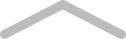# Short Book# Chapter 1Log in or Join Tablo to comment on this chapter...

# Where is the light

Where is the light that was suppose to shine in my world

Where is the birds that were suppose to sing at my window

Where is the wind that’s suppose to whisper peace in my ears

Where is the light that was suppose to shown me these imaginations exist

Where is the light that’s suppose to guide me

Where is the light that’s suppose to cry out to me to pull me out the dark

The light, the light is here, somewhere, the light is in me, but with being torn down used repeatedly the light died but aren’t there suppose to be another light to replace

Where is the lightLog in or Join Tablo to comment on this chapter...
~
69 mins to go